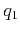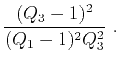On anelliptic approximations for qP velocities in TI and orthorhombic mediaNext: Examples Up: Proposed Approximations Previous: Proposed Approximations

### Moveout approximation

The group-velocity approximation in equation 24 can be easily converted into the corresponding moveout equation using the relationship between offset (), vertical distance (), and total reflection traveltime () given by(31)

whereis the depth of the reflector,is the vertical two-way reflection traveltime, and V() is the approximated group velocity. The moveout equation corresponding to equation 24 is thus,(32)

wheredenotes the NMO-velocity (Alkhalifah and Tsvankin, 1995) and is given by(33)denotes the hyperbolic part of the reflection traveltime squared and is given by(34)

Assuming a particular media type and using a linear relationship betweenand, we reduce the number of independent moveout parameters in the similar manner. However, note that(equations 29) and(equation 30) also depend onand. Therefore, to effectively reduce the number of parameters in the moveout approximation (equation 32) to three, we suggest, as an approximation, to adoptonly for equations 29 and 30, which lead to(35)

As a result, the moveout approximation depends on,, and.

For small offsets, the Taylor expansion of equation 32 is(36)

which reduces to the expression given by Fomel (2004) by setting. The asymptote of this expression for unbounded offsetis given by(37)

which is the horizontal velocity squared.

In the Muir-Dellinger notation, another nonhyperbolic moveout approximation, the generalized nonhyperbolic moveout approximation (Stovas, 2010; Fomel and Stovas, 2010) can be expressed as(38)If the empirical assumption of, or equivalently acoustic approximation is used, equation 38 reduces to the moveout approximation of Fomel (2004).On anelliptic approximations for qP velocities in TI and orthorhombic mediaNext: Examples Up: Proposed Approximations Previous: Proposed Approximations

2017-04-14